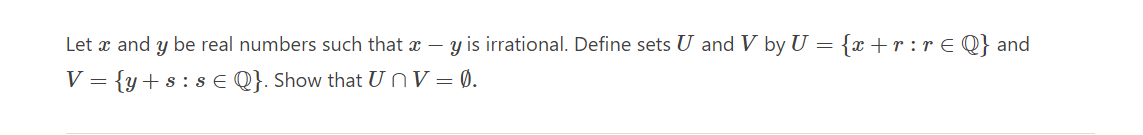# (Solved): Let $$x$$ and $$y$$ be real numbers such that $$x-y$$ is irrational. Define sets $$U$$ and ...Let $$x$$ and $$y$$ be real numbers such that $$x-y$$ is irrational. Define sets $$U$$ and $$V$$ by $$U=\{x+r: r \in \mathbb{Q}\}$$ and $$V=\{y+s: s \in \mathbb{Q}\}$$. Show that $$U \cap V=\emptyset$$.

We have an Answer from Expert